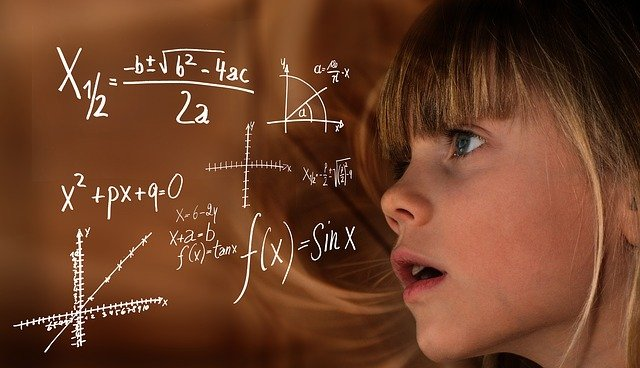## Physics for XIPhysics is one of the most fundamental discipline of science its main goal is to understand how the universe behave. Physics is about asking fundamental questions and trying to answer them by observing and experimenting. This course is designed for XI class students to enhance their theoretical understanding as well as problem solving skills, that will help in writing their exams and strong the fundamentals to crack a competitive exam.

## course syllabus• 1.1 What is physics ?
• 1.2 Scope and excitement of physics
• 1.3 Physics, technology and society
• 1.4 Fundamental forces in nature
• 1.5 Nature of physical laws
• 2.1 Introduction
• 2.2 The international system of units
• 2.3 Measurement of length
• 2.4 Measurement of mass
• 2.5 Measurement of time
• 2.6 Accuracy, precision of instruments and errors in measurement
• 2.7 Significant figures
• 2.8 Dimensions of physical quantities
• 2.9 Dimensional formulae and dimensional equations
• 2.10 Dimensional analysis and its applications
• 3.1 Introduction
• 3.2 Position, path length and displacement
• 3.3 Average velocity and average speed
• 3.4 Instantaneous velocity and speed
• 3.5 Acceleration
• 3.6 Kinematic equations for uniformly accelerated motion
• 3.7 Relative velocity
• 4.1 Introduction
• 4.2 Scalars and vectors
• 4.3 Multiplication of vectors by real numbers
• 4.4 Addition and subtraction of vectors – graphical method
• 4.5 Resolution of vectors
• 4.6 Vector addition – analytical method
• 4.7 Motion in a plane
• 4.8 Motion in a plane with constant acceleration
• 4.9 Relative velocity in two dimensions
• 4.10 Projectile motion
• 4.11 Uniform circular motion
• 5.1 Introduction
• 5.2 Aristotle’s fallacy
• 5.3 The law of inertia
• 5.4 Newton’s first law of motion
• 5.5 Newton’s second law of motion
• 5.6 Newton’s third law of motion
• 5.7 Conservation of momentum
• 5.8 Equilibrium of a particle
• 5.9 Common forces in mechanics
• 5.10 Circular motion
• 5.11 Solving problems in mechanics
• 6.1 Introduction
• 6.2 Notions of work and kinetic energy : The work-energy theorem
• 6.3 Work
• 6.4 Kinetic energy
• 6.5 Work done by a variable force
• 6.6 The work-energy theorem for a variable force
• 6.7 The concept of potential energy
• 6.8 The conservation of mechanical energy
• 6.9 The potential energy of a spring 123
• 6.10 Various forms of energy : the law of conservation of energy
• 6.11 Power
• 6.12 Collisions
• 7.1 Introduction
• 7.2 Centre of mass
• 7.3 Motion of centre of mass
• 7.4 Linear momentum of a system of particles
• 7.5 Vector product of two vectors
• 7.6 Angular velocity and its relation with linear velocity
• 7.7 Torque and angular momentum
• 7.8 Equilibrium of a rigid body
• 7.9 Moment of inertia
• 7.10 Theorems of perpendicular and parallel axes
• 7.11 Kinematics of rotational motion about a fixed axis 167
• 7.12 Dynamics of rotational motion about a fixed axis 169
• 7.13 Angular momentum in case of rotations about a fixed axis 171
• 7.14 Rolling motion 173
• 8.1 Introduction
• 8.2 Kepler’s laws
• 8.3 Universal law of gravitation
• 8.4 The gravitational constant
• 8.5 Acceleration due to gravity of the earth
• 8.6 Acceleration due to gravity below and above the surface of earth
• 8.7 Gravitational potential energy
• 8.8 Escape speed
• 8.9 Earth satellite
• 8.10 Energy of an orbiting satellite
• 8.11 Geostationary and polar satellites
• 8.12 Weightlessness
• 9.1 Introduction
• 9.2 Elastic behaviour of solids
• 9.3 Stress and strain
• 9.4 Hooke’s law
• 9.5 Stress-strain curve
• 9.6 Elastic moduli
• 9.7 Applications of elastic behaviour of materials
• 10.1 Introduction
• 10.2 Pressure
• 10.3 Streamline flow
• 10.4 Bernoulli’s principle
• 10.5 Viscosity
• 10.6 Reynolds number
• 10.7 Surface tension
• 11.1 Introduction
• 11.2 Temperature and heat
• 11.3 Measurement of temperature
• 11.4 Ideal-gas equation and absolute temperature
• 11.5 Thermal expansion
• 11.6 Specific heat capacity
• 11.7 Calorimetry
• 11.8 Change of state
• 11.9 Heat transfer
• 11.10 Newton’s law of cooling
• 12.1 Introduction
• 12.2 Thermal equilibrium
• 12.3 Zeroth law of thermodynamics
• 12.4 Heat, internal energy and work
• 12.5 First law of thermodynamics
• 12.6 Specific heat capacity 308
• 12.7 Thermodynamic state variables and equation of state 309
• 12.8 Thermodynamic processes 310
• 12.9 Heat engines 313
• 12.10 Refrigerators and heat pumps 313
• 12.11 Second law of thermodynamics 314
• 12.12 Reversible and irreversible processes 315
• 12.13 Carnot engine 316
• 13.1 Introduction
• 13.2 Molecular nature of matter
• 13.3 Behaviour of gases
• 13.4 Kinetic theory of an ideal gas
• 13.5 Law of equipartition of energy
• 13.6 Specific heat capacity
• 13.7 Mean free path
• 14.1 Introduction
• 14.2 Periodic and oscilatory motions
• 14.3 Simple harmonic motion
• 14.4 Simple harmonic motion and uniform circular motion
• 14.5 Velocity and acceleration in simple harmonic motion
• 14.6 Force law for simple harmonic motion
• 14.7 Energy in simple harmonic motion
• 14.8 Some systems executing Simple Harmonic Motion
• 14.9 Damped simple harmonic motion
• 14.10 Forced oscillations and resonance
• 15.1 Introduction
• 15.2 Transverse and longitudinal waves
• 15.3 Displacement relation in a progressive wave
• 15.4 The speed of a travelling wave
• 15.5 The principle of superposition of waves
• 15.6 Reflection of waves
• 15.7 Beats
• 15.8 Doppler effect